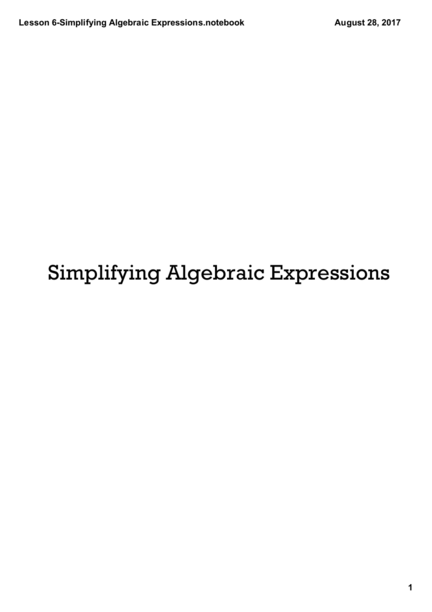# Simplifying Algebraic ExpressionsContributed by:This pdf includes the following topics:-
Algebraic expression
Like Terms
Unlike Terms
The Commutative Property
The Associative Property
The Distributive Property
1. Lesson 6­Simplifying Algebraic Expressions.notebook August 28, 2017
Simplifying Algebraic Expressions
1
2. Lesson 6­Simplifying Algebraic Expressions.notebook August 28, 2017
Algebraic expression: a combination of
numbers, letters (variables), and operation
symbols
Examples:
4x n+7 3y ­ 5 ­ x
How do you read each of the expressions
above?
2
3. Lesson 6­Simplifying Algebraic Expressions.notebook August 28, 2017
The addends of an algebraic expression are
called the terms of the expression.
x+3
2 terms
3y2 ­ 6y + 4
3 terms
constant: a number without a variable
variable term: a term with a variable
coefficient: the number in front of a variable
3
4. Lesson 6­Simplifying Algebraic Expressions.notebook August 28, 2017
LIKE TERMS have the same variable factors
but can have different coefficients
Like Terms: Unlike Terms:
3x, ­4x 5x, x2
­6y, 2y, y 7x, 7y
4
5. Lesson 6­Simplifying Algebraic Expressions.notebook August 28, 2017
True or False? The following terms are like
terms.
1. 3x & 3y
2. 5x & ­9x
3. 2x2 & 2y2
4. ­7xz2 & 3z2x
5
6. Lesson 6­Simplifying Algebraic Expressions.notebook August 28, 2017
Like terms can be combined when using the
properties of numbers. The properties help
us to simplify expressions.
An algebraic expression is simplified when
all like terms have been combined.
6
7. Lesson 6­Simplifying Algebraic Expressions.notebook August 28, 2017
The Commutative Property:
a+b=b+a
Multiplication:
ab = ba
*The ORDER of adding or multiplying two
numbers can be changed without changing
their sum or product*
7
8. Lesson 6­Simplifying Algebraic Expressions.notebook August 28, 2017
EXAMPLES:
1. 2 + 3 = 3 + 2
2. 7(9) = 9(7)
8
9. Lesson 6­Simplifying Algebraic Expressions.notebook August 28, 2017
The Associative Property:
(a + b) + c = a + (b + c)
Multiplication:
(ab)c = a(bc)
The GROUPING of numbers in addition or
multiplication can be changed without
changing their sum or product.
9
10. Lesson 6­Simplifying Algebraic Expressions.notebook August 28, 2017
EXAMPLES:
1. (1 + 8) + 10 = 1 + (8 + 10)
2. (4 2) 3 = 4 (2 3)
10
11. Lesson 6­Simplifying Algebraic Expressions.notebook August 28, 2017
The Distributive Property:
If a, b, and c are numbers, then...
a(b + c) = ab + ac a(b ­ c) = ab ­ ac
or or
(a + b)c = ac + bc (a ­ b)c = ac ­ bc
11
12. Lesson 6­Simplifying Algebraic Expressions.notebook August 28, 2017
EXAMPLES:
1. 2(x + 3)
2. (y ­ 1)5
3. (7 + 2)x
12
13. Lesson 6­Simplifying Algebraic Expressions.notebook August 28, 2017
Use the properties of numbers for addition to
simplify each expression by combining like
terms.
1. 4x + 6x 4. 2y ­ 6 + 4y + 8
2. y ­ 5y 5. 2x ­ 5 + 3y + 4x ­ 10y + 11
3. 3x2 + 5x2 ­ 2
13
14. Lesson 6­Simplifying Algebraic Expressions.notebook August 28, 2017
Use the properties of numbers for multiplication
to simplify each expression by combining like
terms.
1. 5(3y) 4. ­3(5a + 2)
2. ­2(4x) 5. 8(x ­ 4)
3. 5(x + 4)
14